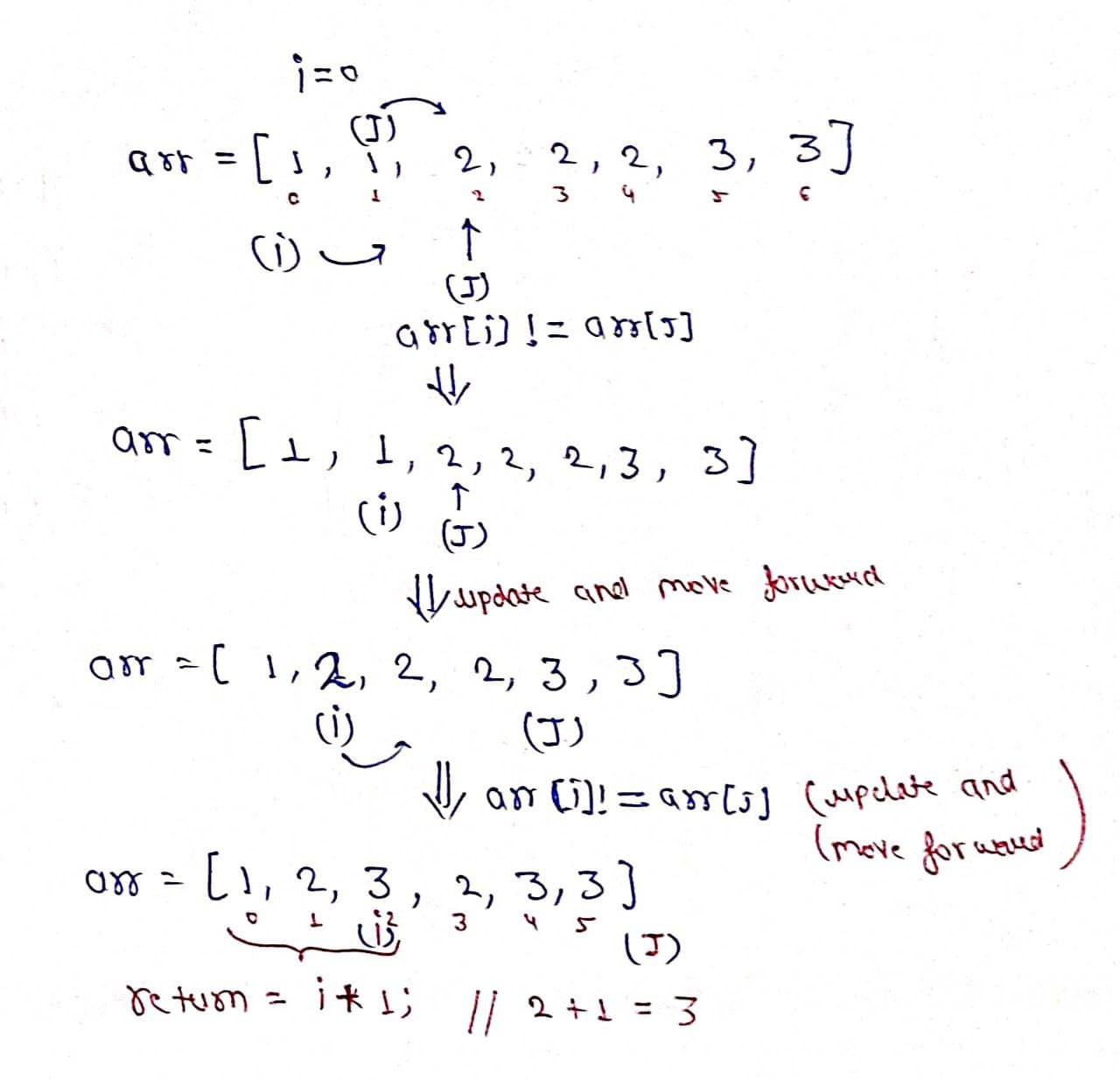# Remove Duplicates in-place from Sorted Array

Problem Statement: Given an integer array sorted in non-decreasing order, remove the duplicates in place such that each unique element appears only once. The relative order of the elements should be kept the same.

If there are k elements after removing the duplicates, then the first k elements of the array should hold the final result. It does not matter what you leave beyond the first k elements.

Note: Return k after placing the final result in the first k slots of the array.

Examples:

```Example 1:

Input: arr[1,1,2,2,2,3,3]

Output: arr[1,2,3,_,_,_,_]

Explanation: Total number of unique elements are 3, i.e[1,2,3] and Therefore return 3 after assigning [1,2,3] in the beginning of the array.

Example 2:

Input: arr[1,1,1,2,2,3,3,3,3,4,4]

Output: arr[1,2,3,4,_,_,_,_,_,_,_]

Explanation: Total number of unique elements are 4, i.e[1,2,3,4] and Therefore return 4 after assigning [1,2,3,4] in the beginning of the array.
```

### Solution

Disclaimer: Don’t jump directly to the solution, try it out yourself first.

Solution 1: Brute Force

Intuition: We have to think of a data structure that does not store duplicate elements. So can we use a Hash set? Yes! We can. As we know HashSet only stores unique elements.

Approach:

• Declare a HashSet.
• Run a for loop from starting to the end.
• Put every element of the array in the set.
• Store size of the set in a variable K.
• Now put all elements of the set in the array from the starting of the array.
• Return K.

Code:

## C++ Code

``````#include<bits/stdc++.h>

using namespace std;
int removeDuplicates(int arr[], int n) {
set < int > set;
for (int i = 0; i < n; i++) {
set.insert(arr[i]);
}
int k = set.size();
int j = 0;
for (int x: set) {
arr[j++] = x;
}
return k;
}
int main() {
int arr[] = {1,1,2,2,2,3,3};
int n = sizeof(arr)/sizeof(arr);
int k = removeDuplicates(arr, n);
cout << "The array after removing duplicate elements is " << endl;
for (int i = 0; i < k; i++) {
cout << arr[i] << " ";
}
}``````

Output: The array after removing duplicate elements is 1 2 3

Time complexity: O(n*log(n))+O(n)

Space Complexity: O(n)

## Java Code

``````import java.util.*;
public class Main {
public static void main(String[] args) {
int arr[] = {1,1,2,2,2,3,3};
int k = removeDuplicates(arr);
System.out.println("The array after removing duplicate elements is ");
for (int i = 0; i < k; i++) {
System.out.print(arr[i] + " ");
}
}
static int removeDuplicates(int[] arr) {
HashSet < Integer > set = new HashSet < > ();
for (int i = 0; i < arr.length; i++) {
}
int k = set.size();
int j = 0;
for (int x: set) {
arr[j++] = x;
}
return k;
}
}``````

Output: The array after removing duplicate elements is 1 2 3

Time complexity: O(n*log(n))+O(n)

Space Complexity: O(n)

Solution 2: Two pointers

Intuition: We can think of using two pointers ‘i’ and ‘j’, we move ‘j’ till we don’t get a number arr[j] which is different from arr[i]. As we got a unique number we will increase the i pointer and update its value by arr[j].

Approach:

• Take a variable i as 0;
• Use a for loop by using a variable ‘j’ from 1 to length of the array.
• If arr[j] != arr[i], increase ‘i’ and update arr[i] == arr[j].
•  After completion of the loop return i+1, i.e size of the array of unique elements.Code:

## C++ Code

``````#include<bits/stdc++.h>

using namespace std;
int removeDuplicates(int arr[], int n)
{
int i = 0;
for (int j = 1; j < n; j++) {
if (arr[i] != arr[j]) {
i++;
arr[i] = arr[j];
}
}
return i + 1;
}
int main() {
int arr[] = {1,1,2,2,2,3,3};
int n = sizeof(arr)/sizeof(arr);
int k = removeDuplicates(arr, n);
cout << "The array after removing duplicate elements is " << endl;
for (int i = 0; i < k; i++) {
cout << arr[i] << " ";
}
}``````

Output:

The array after removing duplicate elements is 1 2 3

Time complexity: O(n)

Space Complexity: O(1)

## Java Code

``````import java.util.*;
public class Main {
public static void main(String[] args) {
int arr[] = {1,1,2,2,2,3,3};
int k = removeDuplicates(arr);
System.out.println("The array after removing duplicate elements is ");
for (int i = 0; i < k; i++) {
System.out.print(arr[i] + " ");
}
}
static int removeDuplicates(int[] arr) {
int i = 0;
for (int j = 1; j < arr.length; j++) {
if (arr[i] != arr[j]) {
i++;
arr[i] = arr[j];
}
}
return i + 1;
}
}``````

Output:

The array after removing duplicate elements is 1 2 3

Time complexity: O(n)

Space Complexity: O(1)

Special thanks to Prashant Sahu for contributing to this article on takeUforward. If you also wish to share your knowledge with the takeUforward fam, please check out this article